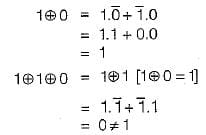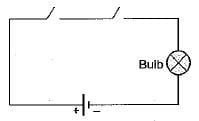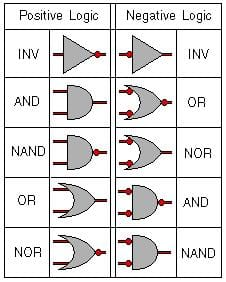# Test: Boolean Algebra & Logic Gates- 1

## 10 Questions MCQ Test Question Bank for GATE Computer Science Engineering | Test: Boolean Algebra & Logic Gates- 1

Description
Attempt Test: Boolean Algebra & Logic Gates- 1 | 10 questions in 30 minutes | Mock test for Computer Science Engineering (CSE) preparation | Free important questions MCQ to study Question Bank for GATE Computer Science Engineering for Computer Science Engineering (CSE) Exam | Download free PDF with solutions
QUESTION: 1

Solution:
QUESTION: 2

Solution:
QUESTION: 3

### Which of the following logic expression is incorrect?

Solution:

The expression:Hence, (b) is the correct option.

QUESTION: 4

Let X and Y be the input and Z be the output of a AND gate. The value of Z is given by

Solution:
QUESTION: 5

What logic gate is represented by the circuit shown below:Solution:
QUESTION: 6

The boolean expression A + BC equals

Solution:

A + BC represents the distributive law which can be expanded as A + BC = (A + B) . (A + C)

QUESTION: 7

A positive AND gate is also a negative

Solution:QUESTION: 8

A gate in which all inputs must be low to get a high output is called a/an

Solution:
QUESTION: 9

Logic 1 in +ve logic system is represented by

Solution:
QUESTION: 10

An AND circuit

Solution:Use Code STAYHOME200 and get INR 200 additional OFF Use Coupon Code